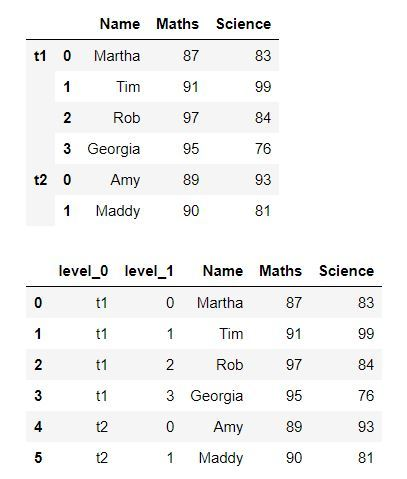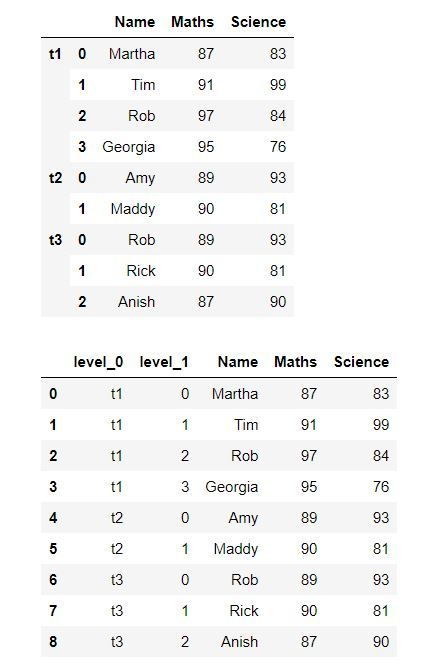# How To Add Identifier Column When Concatenating Pandas dataframes?

• Last Updated : 18 Apr, 2022

We generally want to concat two or more dataframes when working with some data. So, when we concat these dataframes we need to actually want to provide an identifier column in order to identify the concatenated dataframes. In this article, we’ll see with the help of examples of how we can do this.

Example 1:

To add an identifier column, we need to specify the identifiers as a list for the argument “keys” in concat() function, which creates a new multi-indexed dataframe with two dataframes concatenated. Now we’ll use reset_index to convert multi-indexed dataframe to a regular pandas dataframe.

## Python3

 `import` `pandas as pd``import` `numpy as np`  `dict` `=` `{``'Name'``:[``'Martha'``, ``'Tim'``, ``'Rob'``, ``'Georgia'``],``        ``'Maths'``:[``87``, ``91``, ``97``, ``95``],``        ``'Science'``:[``83``, ``99``, ``84``, ``76``]``       ``}` `df1 ``=` `pd.DataFrame(``dict``)` `dict` `=` `{``'Name'``:[``'Amy'``, ``'Maddy'``],``        ``'Maths'``:[``89``, ``90``],``        ``'Science'``:[``93``, ``81``]``       ``}` `df2 ``=` `pd.DataFrame(``dict``)` `# Concatenating two dataframes``df ``=` `pd.concat([df1,df2],keys``=``[``'t1'``, ``'t2'``])``display(df)` `df ``=` `pd.concat([df1,df2], keys``=``[``'t1'``, ``'t2'``]).reset_index()``display(df)`

Output:In the output, we can see a column with the identifiers of each dataframe where “t1” represents the first dataframe and “t2” represents the second dataframe.

Example 2:

We can do this similarly for any number of dataframes. In this example, we’ll combine three dataframes.

## Python3

 `import` `pandas as pd``import` `numpy as np`  `dict` `=` `{``'Name'``: [``'Martha'``, ``'Tim'``, ``'Rob'``, ``'Georgia'``],``        ``'Maths'``: [``87``, ``91``, ``97``, ``95``],``        ``'Science'``: [``83``, ``99``, ``84``, ``76``]``        ``}` `df1 ``=` `pd.DataFrame(``dict``)` `dict` `=` `{``'Name'``: [``'Amy'``, ``'Maddy'``],``        ``'Maths'``: [``89``, ``90``],``        ``'Science'``: [``93``, ``81``]``        ``}` `df2 ``=` `pd.DataFrame(``dict``)` `dict` `=` `{``'Name'``: [``'Rob'``, ``'Rick'``, ``'Anish'``],``        ``'Maths'``: [``89``, ``90``, ``87``],``        ``'Science'``: [``93``, ``81``, ``90``]``        ``}` `df3 ``=` `pd.DataFrame(``dict``)` `# Concatenating Dataframes``df ``=` `pd.concat([df1, df2, df3],``               ``keys``=``[``'t1'``, ``'t2'``, ``'t3'``])``display(df)` `df ``=` `pd.concat([df1, df2, df3],``               ``keys``=``[``'t1'``, ``'t2'``, ``'t3'``]).reset_index()``display(df)`

Output:In the output, we can see a column with the identifiers of each dataframe where “t1”, “t2” and “t3” represent first, second and third dataframe respectively.

My Personal Notes arrow_drop_up